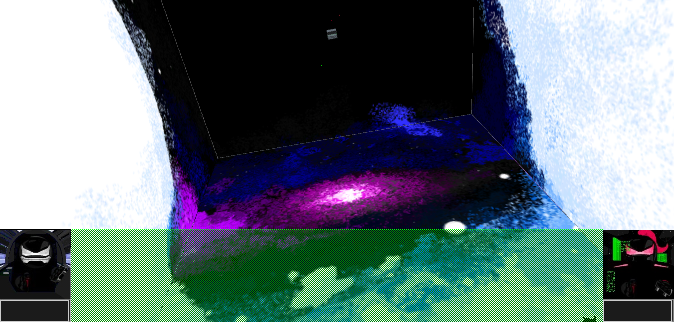# Enter the coordinates  then proceed to step #1

1st Coordinate = (x1, y1, z1) =
(, , )

2nd Coordinate = (x2, y2, z2) =
(, , )Project Jade Red and Cannonballhead:

"Version 3.0.6 Jade Red and Cannonballhead XYZ Axis Coordinates Distance Calculator Calculator Version 3.0.6 Calculator"

# (The coordinates you entered in the window above for the players are now shown in the equation here in step #1) Click each red, green, and blue subtraction equation buttons to show an answer and a color. Then place each answer into the matching color boxes of step #2.

distance = √(( - )2 + ( - )2 + ( - )2)

(x₁ - x₂)=

=
+
(y₁ - y₂)=

=
+
(z₁ - z₂)=

= )

# Step #3

After you have answers in each lime colored box of step #2 you can press this button (place this orange color answer into the orange and purple boxes of step 4)

# Step #4

Distance =
√()
(Orange Box = This is your answer written as a square root. You will need to simplify square root answers when necessary) [example: √12 is the same as 2√3. √6 is not the same as 2√3.]
√() (Click this button to use the √(square root) function)
Distance = The square root of your number = (this is your answer as a number)## Elevation Angles

Examples to use:

• Use the distance you found in the 'XYZ Axis Coordinates Distance Calculator Calculator' as the hypotenuse length.
• A positive form of the green colored answer in step #1 of the XYZ coordinates calculator can be used as the measurement of the distance of the other player's height above or below you. It is a y-axis length and is the axis your Communication Core's Drone Satellite will travel on, in a vertical direction. (If the green answer in step #1 is zero there is no elevation angle to solve for.)
• Insert 2 lengths into a set of yellow and turquoise bordered boxes. Then press the red 'PUSH' button.
• Copy and paste answers into the matching color input boxes. Use the corresponding buttons to provide answers into the next color box.

#### If you know the length opposite the angle and the hypotenuse, use this calculator.

/
(note: Hypotenuse must be longer than the opposite length)

Radians

Degrees

#### If you know the adjacent length and the hypotenuse length, use this calculator.

/
(note: Hypotenuse must be longer than the adjacent length)

Radians

Degrees

#### If you know the length opposite the angle and the adjacent length, use this calculator.

/

Radians

Degrees

``` PREPARE FOR ARC LENGTH MEASUREMENT!!! Arc Length = 2πr(angle° ÷ 360) Surface Area of a Sphere = 4πr2 Volume of a Sphere = (4/3)πr3 Density = mass/volume Circumference of a Circle C = 2πr    =    C = πd The equation of a circle with center (a, b), radius (r), and outer coordinate (x, y) : (x - a)2 + (y - b)2 = r2 The equation of a sphere with center (a, b, c), radius (r), and a coordinate located a distance equal to the radius from center represented as (x, y, z) : ((x - a)2 + (y - b)2 + (z - c)2) = r2 Pythagorean Theorem a2 + b2 = c2 Hz = Hertz ```

# Jade Red and Cannonballhead : Escape Velocity

Jade Red's and Cannonballhead's axis ranges:
X minimum -206265 and maximum 206265
Y minimum -206265 and maximum 206265
Z minimum -206265 and maximum 206265
Players axis ranges are from -206265 to 206265

Jade Red's and Cannonballhead's locations on:
and milliseconds
(The digital time display is stopped time in 24 hour format; hours:minutes:seconds)

X Y Z
Jade Red
Cannonballhead
Coins Red Orange Yellow Green Blue Indigo Violet
0 0 0 0
The coin count starts at 0 every 24 hours starting at midnight.
```60 red      = 1 Coin  //occurs every hour
60 orange   = 1 red  //occurs every minute
1000 yellow = 1 orange  //occurs every second
10 green    = 1 yellow  //occurs every .001 of a second
10 blue     = 1 green  //occurs every .0001 of a second
10 indigo   = 1 blue  //occurs every .00001 of a second
10 violet   = 1 indigo  //occurs every .000001 of a second

```Players Communication Core Color X Y Z
Computer White RGB(255,255,255)
Computer Red RGB(255,0,0) -500 300 400
Computer Orange RGB(255,165,0) -500 300 -400
Computer Yellow RGB(255,255,0) 500 300 -400
Computer Green RGB(255,128,0) 500 -300 400
Computer Blue RGB(0,0,255) -500 -300 400
Computer Indigo RGB(75,0,130) -500 -300 -400
Computer Violet RGB(238,130,238) 500 -300 -400

Movement Simulator version 1.1.1

X

Y

Z

To calculate the speed required, to travel a known distance and time, use this equation:
Distance/Time = Rate

÷ = Rate

To calculate the amount of time, to travel a known distance and speed, use this equation:
Distance/Rate=Time

÷ = Time

To calculate the distance, of a known speed and time, use this equation:
Distance = Rate × Time

× = DistancePASS CODE LOCK SIMULATOR

# Pirate's Dice

• Five of a kind
• Four of a kind
• Full House
• Straight
• Three of a kind
• Two Pair
• One Pair
• High Dice

(Note: As in real dice, after a dice is clicked a second time, the same number can occur again.)

# Jade Red and Cannonballhead : Project Peas and Carrots